# How to Solve Multi-Facts

Multi-Facts puzzles are designed by an award winning teacher in California to be a fun and easy way to help your child study their multiplication facts while building a solid foundation in math that will help them for years to come. These puzzles combine the logical thinking skills and repetition needed for any student to master their multiplication facts.

### How do I solve a Multi-Facts puzzle?

Multi-Facts puzzles have similar rules to the popular Sudoku puzzles, so if you know how to solve a Sudoku puzzle, you already know the rules for solving a Multi-Facts puzzle. However, if you are unfamiliar with Sudoku puzzles, all you need to understand Multi-Facts puzzles is one definition and three basic rules.

Definition - A Multiple is the answer to a multiplication problem. i.e. 4,8,12,16,20,24,28,32 and 36 are all multiples of the number 4. Multi-Facts puzzles repeatedly use the first 9 multiples of a given root number.

The 3 rules used to solve Multi-Facts puzzles.

Rule #1 - Each Column can only have 1 multiple for each number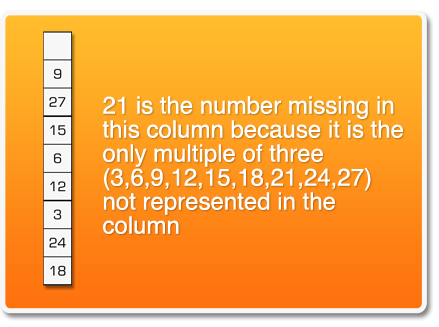Rule #2 - Each Row can only have 1 multiple for each number.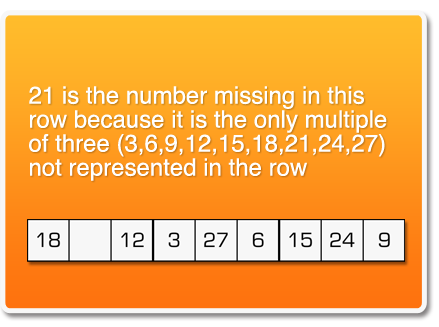Rule #3 - Each 3 x 3 grid can only have 1 multiple for each number.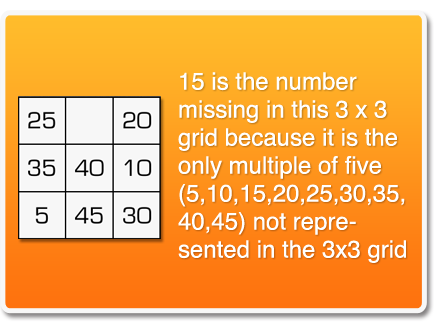### Why Choose Multi-Facts?

Why is this so hard for some students? Students and teachers must recognize that learning the multiplication facts is a memorization task. It does not reflect a person's math ability. Learn more.

If this is not something tweet worthy, then we don't know what is. How can you not share this?

The Multi-Facts iPad App is LIVE on the iTunes App Store. If you have a child and an iPad, go get the App!! If you don't have an iPad, what are you waiting for?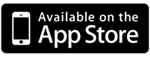### Free Multiplications Tables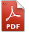This 9x12 multiplication chart is a perfect tool to help students with all Multi-Facts puzzles. Some teachers require that students learn their multiples up to 12 so we created a chart that includes these multipliers. Download Here

### How to Solve Multi-Facts

Multi-Facts puzzles have similar rules to the popular Sudoku puzzles. If you are unfamiliar with Sudoku puzzles, all you need to understand Multi-Facts puzzles is one definition and three basic rules. Get more info.